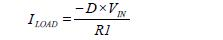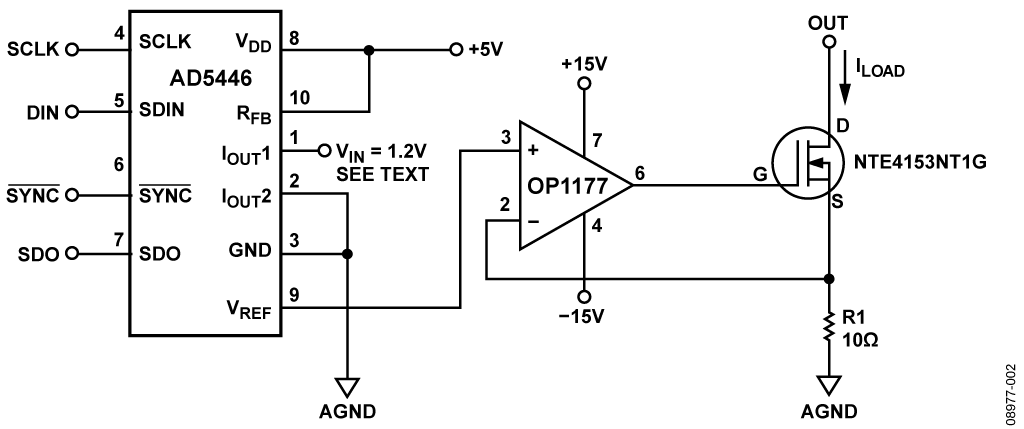# Обзор

## Ресурсы проектирования

### Драйверы устройств

ПО (код на С и/или FPGA) для связи с цифровым интерфейсом компонента.

## Особенности и преимущества

• Programmable current output
• Multiple configurations for reverse and bi-directional modes

# Circuit Function & Benefits

Digitally controlled current sources are critical functions in a variety of applications, such as power management, solenoid control, motor control, impedance measurement, sensor excitation, and pulse oximetry. Here we describe three current sources with serial interface digital control using DACs, op amps, and MOSFET transistors.

The DACs selected are high resolution (14- or 16-bit), low power CMOS with standard serial interfaces. The AD5543 16-bit DAC is packaged in ultracompact (3 mm × 4.7 mm) 8-lead MSOP and 8-lead SOIC packages. The AD5446 14-bit DAC is available in a small 10-lead MSOP package. The two DACs are both compatible with most DSP interface standards and also SPI, QSPI, and MICROWIRE. The external reference voltage input allows many output level variations, up to 10 V.

The combination of parts represents industry-leading small PC board area, low cost, and high resolution. The three designs offer low risk solutions and use industry-standard parts.

# Описание схемы

All three circuits require a single 5 V supply for the DACs and ±15 V supplies for the op amps. Some circuits may need an accurate external voltage reference (see MT-087 Tutorial).

Each circuit contains two stages. The first stage is the input stage, composed of the DAC and an op amp. The second stage is an N-channel MOSFET transistor output stage (Figure 1 and Figure 2), which supplies the current in response to the digital word sent to the system.

The input stage of the circuit, shown in Figure 1, is composed of a current output DAC (AD5446) and its op amp (AD8510). It provides the conversion of the command word and drives the transistor. It also modulates the voltage applied to the single resistor. The command word is sent via an SPI interface.Figure 1. Current Source Using a Current Output DAC (All Connections and Decoupling Not Shown)

The output stage is composed of an N-channel MOSFET transistor (NTE4153N), which can provide more current than the output of the op amp and a single resistor. The single resistor, R1, produces the current with the voltage applied to its pins. The transistor regulates this current.Where D is the fractional representation of the digital word loaded into the DAC. However, RDAC >> R1, (RDAC is nominally 9 kΩ) so the load current can be approximated asWith R1 = 100 Ω and VIN = −5 V, ILOAD is programmable from 0 mA to 50 mA with a resolution of 3 μA (1 LSB at 14 bits). The output compliance voltage is approximately 20 V and is limited by the breakdown voltage of the MOSFET transistor. The ADR425 is an ideal 5 V low power precision reference for this circuit, but its output must be inverted with an additional op amp to generate the −5 V reference.

The circuit shown in Figure 2 also uses the AD5446 DAC. However, in this case the DAC is used in the reverse or voltage mode, which provides a voltage output by using a 1.2 V voltage reference such as the ADR512.Figure 2. Current Source Using a Current Output DAC Connected in the “Reverse” Voltage Mode (All Connections and Decoupling Not Shown)

The DAC output voltage range on Pin 9 varies from 0 V to 1.2 V. See the AD5446 data sheet for more details on the reverse voltage mode of operation.

The op amp used in this case is the OP1177. It is a high precision and very low offset device (60 μV maximum). Low offset voltage is essential when the DAC is used in voltage output mode because of the reduced signal swing.

The N-channel MOSFET transistor in conjunction with the op amp makes a high current output follower circuit.

The negative feedback from the source pin of the transistor to the op amp input regulates the value of the current through the R1 resistor.With R1=10 Ω and VIN = 1.2 V, ILOAD can be programmed from 0 mA to 120 mA with a resolution of 7 μA (1 LSB @ 14 bits).

The third circuit, shown in Figure 3, uses an AD5543 16-bit DAC as the input and a Howland current pump circuit as the output stage. Howland current pumps have two advantages over MOSFET outputs: high output impedance and the ability to provide bipolar output currents. Usually, to improve stability, the circuit is symmetrical. Therefore R1 = R1', R2 = R2', and R3 = R3'.Figure 3. Bipolar Current Source Based on the Howland Current Source (All Connections and Decoupling Not Shown)

The load current is (see the AN-843 Application Note for derivation)With R1 = 150 kΩ, R2 = 15 kΩ, R3 = 50 Ω, and VIN = 5 V, ILOAD is programmable from 0 mA to 20 mA with a resolution of 300 nA (1 LSB @ 16 bits), and the circuit has a very high output impedance.

Excellent layout, grounding and decoupling techniques must be used in all three circuits in order to separate correctly DACs and op amps and to achieve the desired performances. (See MT-031 and MT-101 tutorial).

# Основные варианты исполнения

In both circuits, other voltage references can be used to get more or less current output range (see Voltage Reference Selection and Evaluation Wizard). Note that a positive reference voltage input generates a negative output current because of the DAC architecture. Although a wide variety of DACs can be used to optimize the design for speed, precision, and so on, CMOS current output DACs such as the AD5543 and AD5446 give more flexibility and provide low risk solutions.

Regarding the op amps, if you have a relatively small output signal range, CMOS amplifiers should work fine. If you want high input impedance, FET input op amps are good choices. In either case, precision amplifiers are required to maintain 14-bit to 16-bit precision.